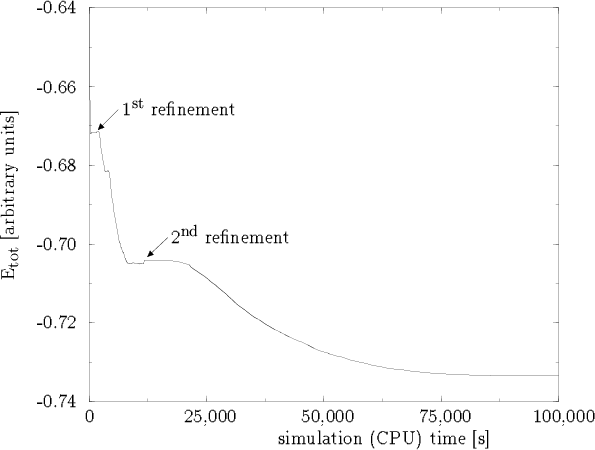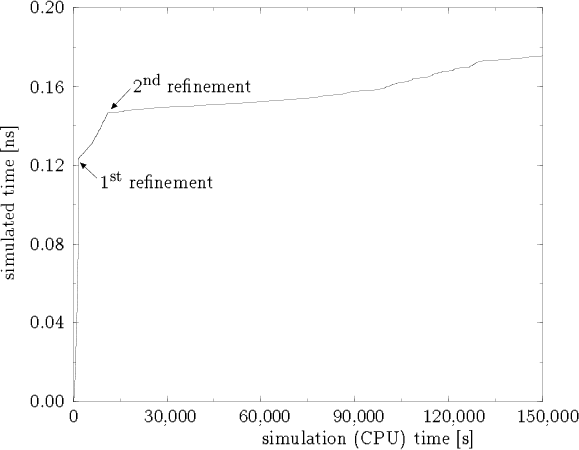Next: Conclusion Up: Application Previous: Hard magnetic cube

## Simulation results

The total energy stored in the magnetization of the cube as a function of the simulation (CPU) time (i.e. the time necessary for the simulation) is shown in figure 7.

During the first 320 seconds the total energy decreases very fast and reaches a minimum value of about -0.672. Then it oscillates slightly and remains stable after 1,000 seconds. Another 640 seconds later the finite element mesh is refined locally as shown in figure 5, but this could have already been done 1,000 seconds after the beginning of the simulation when the total energy remained constant. Immediately the total energy decreases again. The finer mesh permits a more accurate simulation and a more realistic magnetization, which leads to a lower total energy. After a simulation time of 8,000 seconds it arrives at a minimum of -0.705.

The second refinement is applied after 11,700 seconds. The new magnetization vectors originating from the inserted vertices and the interpolation between their neighbours cause a slightly higher total energy, which can be observed as a small step in figure 7. Resuming the simulation, the total energy remains constant at first. After 20,000 seconds it decreases monotonously to its final value of -0.73374. It is reached after 150,000 secondsAnother interesting aspect is the relation between the simulation time and the simulated time shown in figure 8. It indicates how much time and computing power are necessary for the simulation.

Within some seconds of computing time a simulated time of 0.12 ns has passed. There are two reasons for this behaviour: First, the finite element grid consists of 125 vertices and 384 elements only, which makes the calculations quite simple and fast. Secondly the magnetization and the total energy reach very quickly constant values. Therefore the time step resulting from the discretization of time can be enlarged leading to a rapid development of the simulated time.

After the first local refinement the grid consists of 318 vertices and 1,312 elements. Consequently the simulation becomes more complicated and slower. Finally, 1,774 vertices and 8,867 elements assemble the finite element grid.

Yet, it is not possible to decide if the domain wall is of Bloch or Néel type. Some estimated 50,000 elements would be necessary to find the right answer for this hard magnetic cube.Next: Conclusion Up: Application Previous: Hard magnetic cube
Werner Scholz
12/19/1997